Published: 18 September 2015

# Environmental vibration resonances of precise bearing platform built on soft soils

Ping Xu1
Xiaohui Yuan2
1Department of Transportation Engineering, Zhengzhou University, Zhengzhou 450001, P. R. China
2School of Civil and Engineering and Communication, North China University of Water Resources and Electric Power, Zhengzhou 450011, P. R. China
Corresponding Author:
Ping Xu
Views 25

#### Abstract

Environmental vibrations generated by vehicle traffic, rail transition, pile driving, explosion, construction and machine can affect human being’s life and work, destroy building structures and influence instrumentation equipment service life and performance. The active vibration isolation mechanism was given and the range of the isolator parameters are discussed that can obtain the ideal vibration isolation effects. One bearing platform has been designed with active vibration isolation system, the frequency of the ground floor and bearing platform are 4.5 Hz and 7.5-11.5 Hz, and the parameters of the isolators and calculated isolation coefficient all meet with the specifications, but the resonances of the bearing platform caused by the environmental vibrations still take place. The geotechnical conditions under the bearing platform reveal that the water table is about 4.0 m and silt clay with 4.6 m thick exists at the position of 5.7 m from the ground surface, and so the upper soils can easily transmit vibrations and cause the resonances. The soft soils were reinforced with concrete piles and the whole stiffness of the soils was enlarged, and the resonances are thoroughly eliminated, which provides some references for the vibration isolation design at the fields with high water table and soft soils.

## 1. Introduction

With the rapid development of science and technology, modern machining industry (such as optical industry, photosensitive chemical industry, aerospace and semiconductor industry) has entered the era of precision and ultra precision. Large amounts of engineering practices show that the production precision of precise and ultra precise instruments are affected not only by the instrument itself but also the surrounding vibrations such as ground natural vibration, temperature and electromagnetic interference. Sensitive instruments are used in the biological sciences, electronic optics, precision machining, physical and chemical experiments and studies, and good vibration control can reduce the influence of vibrations on instrumentation equipment service life and performance. And so it is an important task to isolate the environmental vibrations that may affect the high precision equipment, instruments and meters.

Before designing vibration isolation platform system for the precision and ultra precision instrument, various complex environmental vibrations are usually considered such mainly the ground vibrations that caused by vehicle traffic, rail transition, pile driving, explosion, construction and machine[1-8]. Environment vibrations mainly includes: the earth pulse ground vibration frequency is 0.0-1.0 Hz; the self vibration frequency of skeleton of building, wall and floor is 15.0-25.0 Hz, human moving vibration frequency is 1.0-3.0 Hz, the vibration frequency caused by ventilation pipe, transformer and engine is 6.0-65.0 Hz, and the vibration frequency of the building itself is 10.0-100.0 Hz . Therefore, environment vibrations with frequency of 0.0-100.0 Hz should be considered when designing the vibration isolation system, which are designed to isolate not only the vibrations with higher frequency such as the operation of high precise instruments, the motion of the rail transition and human movement, but also those with lower frequencies such as surrounding voices and air flows.

One precise bearing platform that built on soft soils is taken as an example, the environmental vibration frequency is measured and the resonance of the platform caused by the environmental vibration is analyzed, and concrete piles are constructed under the platform to eliminate the resonance.

## 2. Resonance mechanism

In the resonance mechanism, the machines or equipments and their basements are usually considered with quality but not elasticity, while the isolator with elasticity and damping but not quality, and the isolation platform in one direction can be simplified as vibration isolation system with single degree of freedom and the computation model is shown in Fig. 1, in which $m$ is quality of machine or equipment and its basement, $k$ and $c$ are the rigidity coefficient and viscous damping coefficient of vibration isolator respectively.

Fig. 1Computation model of vibration isolation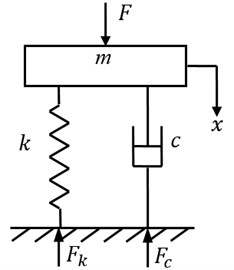a) Active isolation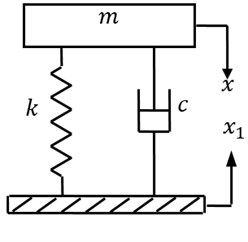b) Passive isolation

The isolation coefficient $K$ is defined as the ratio of the maximum dynamic loads transmitted from the ground to the loads generated by the machine itself :

1
$K=\frac{\sqrt{1+4{\zeta }^{2}{\lambda }^{2}}}{\sqrt{{\left(1-{\lambda }^{2}\right)}^{2}+4{\zeta }^{2}{\lambda }^{2}}},$
2
$\zeta =\frac{c}{{c}_{c}},\lambda =\frac{\omega }{{\omega }_{n}},$

where, $\zeta$ is the ratio of damping coefficient $c$ to the critical damping coefficient ${c}_{c}$, $\lambda$ is the ratio of natural frequency $\omega$ of outer disturbing force to the un-damped natural frequency ${\omega }_{n}$ of the isolation system, and ${c}_{c}$ and ${\omega }_{n}$ are defined as:

3
${c}_{c}=2\sqrt{km},{\omega }_{n}=\sqrt{\frac{k}{m}}.$

Damping coefficient ratio $\zeta$ is valued 0, 0.15, 0.3, 0.5, 1.0 and 2.0, and curves of isolation coefficient $K$ changing with frequency ratio $\lambda$ are obtained as shown in Fig. 2.

Fig. 2 shows that when the frequency ratio $\lambda$ is larger than $\sqrt{\text{2}}$, the isolation coefficient $K$ is less than 1.0, better isolation effects would be obtained, and the relative equation is:

4
$\lambda =\frac{\omega }{{\omega }_{n}}>\sqrt{2},$

and Eq. (4) can be rewritten as follows based on Eq. (3):

5
$\sqrt{\frac{k}{m}}<\frac{\omega }{\sqrt{2}}.$

Eq. (5) shows that there are two ways to obtain better isolation effects: (1) reduce the isolator stiffness, and (2) enlarge the quality of machine or equipment. In the vibration isolation engineering, the frequency ratio is often valued as $\lambda =$ 2.5-5.0.

Fig. 2 also shows that the damping coefficient ratio $\zeta$ is a complex factor that influence the isolation effects: $\zeta$ is smaller and the isolation effect is worse when $\lambda$ is larger than $\sqrt{\text{2}}$, while $\zeta$ is smaller and the isolation effect is better when $\lambda$ is larger than $\sqrt{\text{2}}$. And in the vibration isolation engineering, the damping coefficient ratio is often valued as $\zeta =$ 0.01-0.1.

Fig. 2Curves of isolation coefficient changing with frequency ratio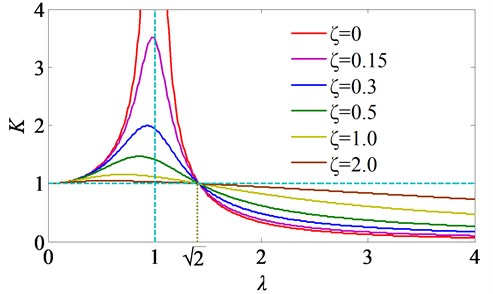## 3. Environmental vibration resonances about precise bearing platform

One precise bearing platform in China P. R. was built on soft soils, where water table is 4.0 m deep from the ground surface and silt clay is 4.6 m thick, and the main mechanical and physical parameters are given in Table 1.

Table 1Main mechanical and physical parameters

 Soil layer Thickness / m Density / kg×m-3 Cohesive strength / kPa Internal friction angle / ° ① Silty clay 5.70 1890 22.2 14.1 ② Silt clay 4.60 1980 4.3 2.5 ③ Clay 4.70 1950 28.5 16.8 ④ Silty clay >30.0 1940 24.3 15.6

The bearing platform has been designed to isolate the environmental vibrations, and the simplified section sketch was given in Fig. 3. The tub was excavated first, then 8 isolators arranged in two rows were put on the tub floor, and finally the bearing platform with concrete basement was installed in the tub.

In the model of the bearing platform, the isolation effects is $\zeta =$0.05, quality of bearing platform and its basement is $m=$ 2.7×104 kg, and the rigidity coefficient of the isolator is $k=$ 2.5×104 N/m, the frequency ratio is $\lambda =$ 2.76-4.23 that calculated from Eqs. (2) and (3), and the two factors of $\zeta$ and $\lambda$ all satisfy with the vibration isolation requirements.

The vertical acceleration of the ground floor around the tub, tub floor and bearing platform were tested when the bearing machine does not work, and the curves of magnitude and frequency are obtained as shown in Fig. 4.

Fig. 4 shows that the tub floor has larger magnitude in the frequency range of 7.5-11.5 Hz, which is generated by the environmental vibration that transmitted in the several meters under the ground surface, the magnitude of the ground floor is slightly less than the tub ground and the magnitude is in the range same to the tub floor, and the bearing platform has larger magnitude with the frequency of 4.5 Hz although the isolators are working. There exists silt clay with 4.6 m thickness under the tub and they are not reinforced, the vibration of the tub floor generated by the environmental vibrations can easily cause the vibration of the bearing platform just like the water wave can easily cause the vibration of the things on the boat.

Fig. 3Vibration isolation model of the bearing platform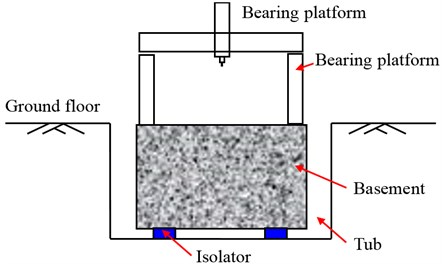In order to eliminate the resonances of the bearing platform caused by the environmental vibrations, the soft soils under the tub must be reinforced, and 4 reinforced concrete filling piles with one pile cap are designed as shown in Fig. 5. The 4 piles are all 0.8 m wide and 20.0 m long, and pile cap is 7.5 m long, 7.5 m wide and 1.0 high.

Fig. 4Curves of displacement magnitude and frequency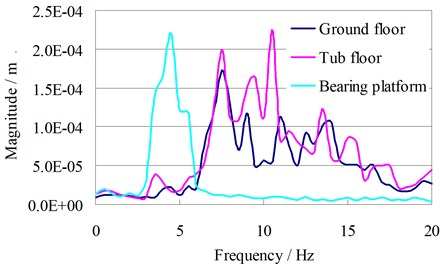Fig. 5Reinforcement of the underground soft soils with pile foundation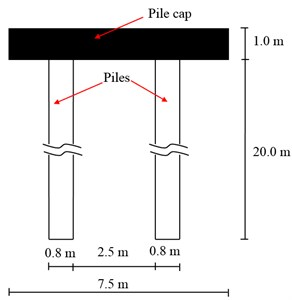Fig. 6Curves of displacement magnitude and frequency after the soft soils are reinforced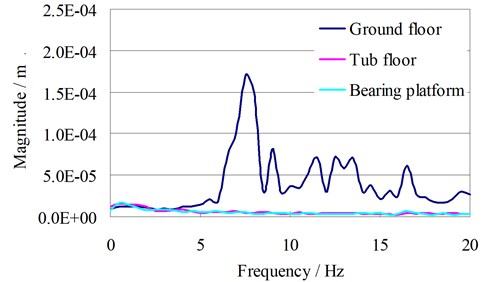After the soft soils under the tub are reinforced, the vertical acceleration of the ground floor, tub floor and bearing platform at the same positions as Fig. 4 were tested again when the bearing machine does not work, and the curves of magnitude and frequency are obtained as shown in Fig. 6.

Fig. 6 shows that after the soft soils were reinforced, the tub floor and bearing platform are effectively isolated from the environmental vibrations, and bearing platform can work with no resonances.

## 4. Conclusions

Although one bearing platform has designed with vibration isolation system with isolators and their parameters meet with the relative specification, the bearing platform has resonances caused by the environmental vibrations. The soft soils under the tub were reinforced with concrete piles and the whole stiffness of the tub was enlarged, and then the resonances caused by the environmental vibrations are thoroughly eliminated.

Accepted
11 August 2015
Published
18 September 2015
SUBJECTS
Mechanical vibrations and applications
Keywords
environmental vibrations
bearing platform
vibration isolation
soft soils
Acknowledgements

The work was supported by National Natural Science Foundation of China (Grant Nos. 51008286, 51278467).